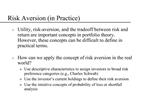DownloadDownload PresentationRisk Aversion in Practice

# Risk Aversion in Practice

Télécharger la présentation## Risk Aversion in Practice

- - - - - - - - - - - - - - - - - - - - - - - - - - - E N D - - - - - - - - - - - - - - - - - - - - - - - - - - -
##### Presentation Transcript

1. Risk Aversion (in Practice) Utility, risk-aversion, and the tradeoff between risk and return are important concepts in portfolio theory. However, these concepts can be difficult to define in practical terms. How can we apply the concept of risk aversion in the real world? Use descriptive characteristics to assign investors to broad risk preference categories (e.g., Charles Schwab) Use the investor�s current holdings to define their risk aversion Use the intuitive concepts of probability of loss or shortfall analysis

2. Probability of Loss One way to describe the risk of a particular investment is to calculate the probability of obtaining a gain or loss of a specified size. Assume that returns are Normally distributed with mean=� and standard deviation=s. In this case, we get: where Z is distributed Normal(0,1). Note that Z can be interpreted as the number of standard deviations the current value is away from the mean. A useful feature of the Normal distribution is that approximately 68.3% of observations lie within one standard deviation of the mean, 95.5% within two std. deviations, and 99.7% within three std. deviations.